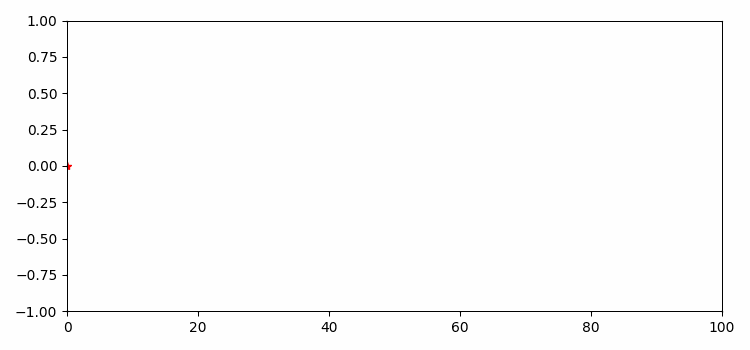# Animation using Matplotlib with subplots and ArtistAnimation

To animate using Matplotlib with subplots and ArtistAnimation, we can take the following steps −

• Set the figure size and adjust the padding between and around the subplots.
• Create a figure and a set of subplots.
• Create a user-defined function, Init, to draw a clear frame.
• Use FuncAnimation to make an animation by repeatedly calling a function *func*.
• Define an animate function to update the data points in FuncArtist class.
• To display the figure, use show() method.

## Example

import numpy as np
import matplotlib.pyplot as plt
from matplotlib.animation import FuncAnimation

plt.rcParams["figure.figsize"] = [7.50, 3.50]
plt.rcParams["figure.autolayout"] = True
fig, ax = plt.subplots()
xdata, ydata = [], []
ln, = plt.plot([], [], 'r*')

def init():
ax.set_xlim(0, 100)
ax.set_ylim(-1, 1)
return ln,
def animate(frame):
xdata.append(frame)
ydata.append(np.sin(frame))
ln.set_data(xdata, ydata)
return ln,

ani = FuncAnimation(fig, animate, init_func=init, blit=True, frames=100)
plt.show()

## Output